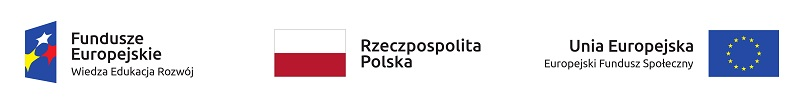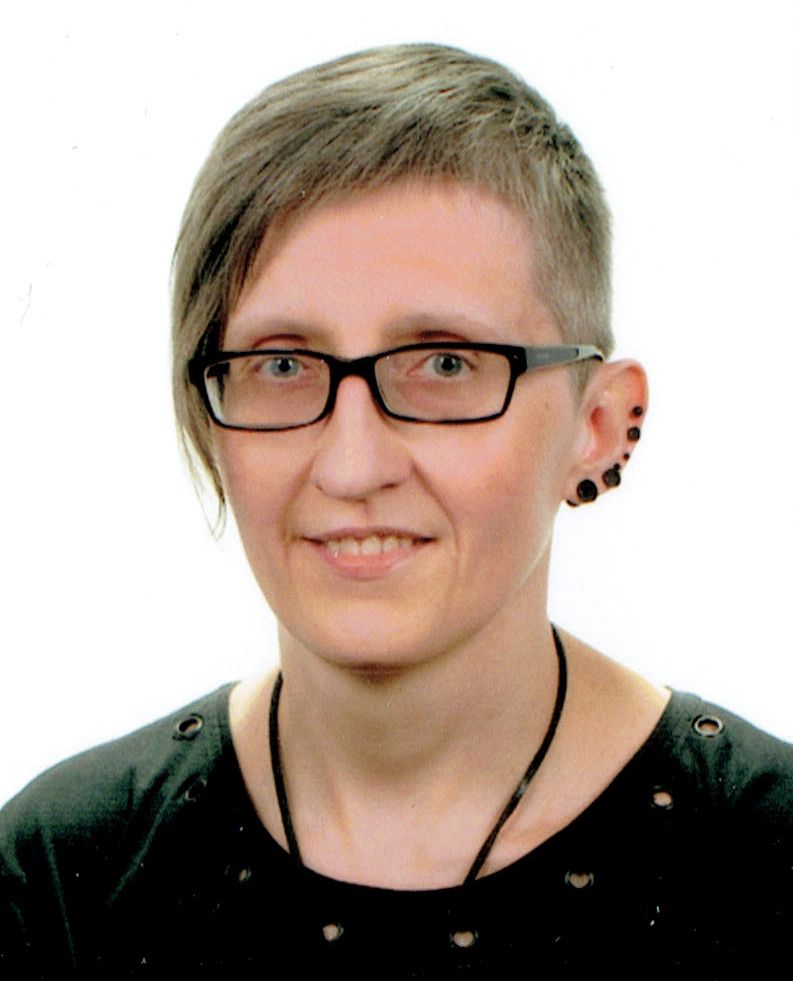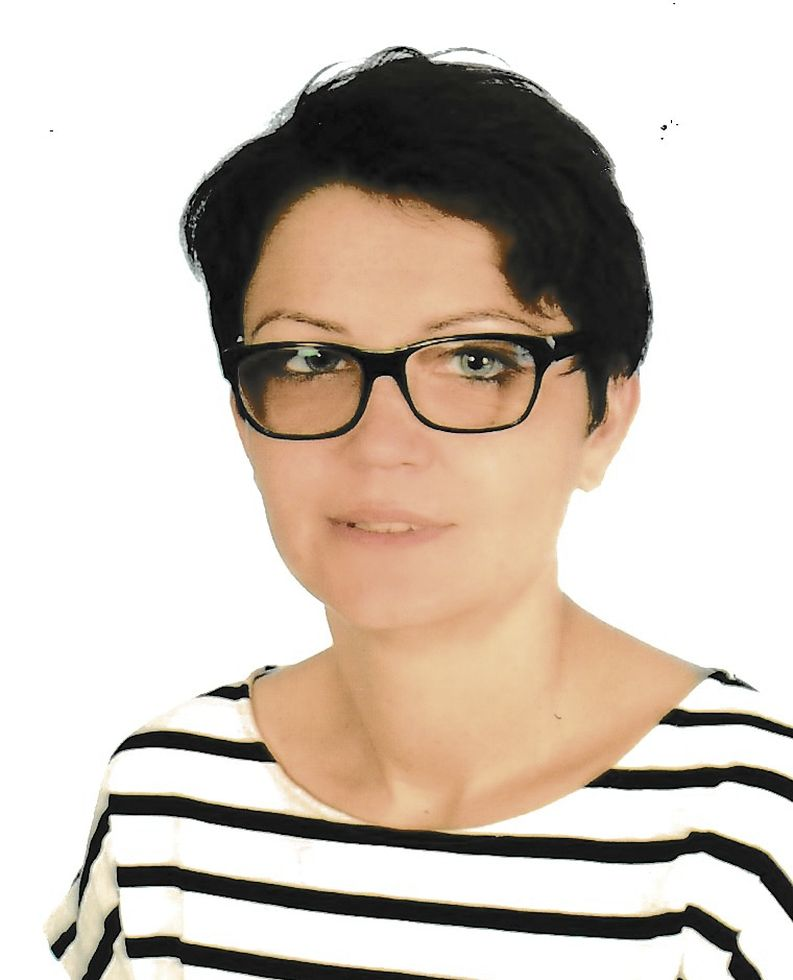## Course DescriptionThe course was created as part of the project "Moocni z matmy – otwarty kurs matematyki" no. POWR.03.01.00-00-W075/18. More on project www.moocni.zut.edu.pl.

Course description

"Strong at maths" is addressed mainly to graduates who intend to study at technical universities, and students of last grades of high schools who, just before the final exams, begin repeating material from mathematics. It will help participants systematize their mathematical knowledge and fill any gaps resulting from the program difference. Thanks to this, it will be easier for the future student to understand new concepts related to higher mathematics. The course contains basic definitions, theorems and formulae in the field of mathematical analysis, algebra, analytical geometry, combinatorics and probability theory. All concepts are supported by numerous examples, tasks solved step by step, drawings and tables as well as GeoGebra applets.

Contents

"Strong at maths" covers most of the issues included in the core curriculum of the standard level for secondary schools. However, there are also concepts that go beyond this level or slightly expand it, but are necessary in the course of studies.

The course consists of 13 thematic chapters:
1. Introduction
2. Functions and their properties
3. Linear function
5. Polynomials
6. Rational function
7. Power function
8. Exponential function
9. Logarithmic function
10. Trigonometric functions
11. Sequences
12. Analytical geometry
13. Elements of combinatorics and probability theory
Course effects
After completing the "Strong at maths" course its participants:
• will have no trouble understanding the symbolic notations of mathematical content used in textbooks, in lessons and lectures,
• will know elementary functions, ways of drawing their graphs and determining their domain, zeros and monotonicity intervals,
• will not have problems with solving equations, inequalities, and even systems of equations in which they occur,
• will be able to efficiently use reduction formulae and trigonometric identities without memorizing them,
• easily distinguishes arithmetic from geometric sequences and calculates the terms of each of them and calculates their sum,
• will be able to perform actions on vectors and check their relative location,
• will easily write the equation of a straight line and a circle with given properties and determine their relative location on a plane, which will allow them to solve equations and inequalities geometrically,
• will know how the permutations of a set, variations with or without repetitions and combinations differ from one another,
• thanks to the multiplication and addition rule will be able to determine the total number of outcomes of a given random experiment,
• will know the scheme of selection of the appropriate combinatorial model that meets the conditions of the task, thanks to which they will calculate the probability of a specific event.
The course

At the beginning of the course, you should read the information contained in the "Preface" module, in particular the user manual and initial diagnosis.

In each of the 13 thematic modules, you should read the theoretical introduction to the given issue. Then you should independently perform the exercises at the end of each unit. They are not scored and can be solved many times until they are not difficult. Because these examples are randomized (you can reset them and receive a task with different parameters), you can practice different versions of the task, view their full solutions and be well prepared for the module test.

At the end of the course, you take the final exam. The final grade of the course is a weighted average of all 13 module tests, which constitute 60% of it, and the final exam, which constitutes 40% of this grade. The course is considered completed if you obtain a final grade with a score of at least 60%.

At the end of the course, you should complete an evaluation questionnaire that will help the course authors adapt it to the needs and expectations of the participants.

After completing the course with a minimum score of 60%, you will receive an electronic certificate within 24 hours.

Prerequisites

The course participant should have basic mathematical and IT knowledge in the field of an eight-year primary or lower secondary school.

Course staffMagdalena Kucharska, PhD
For 25 years she has taught mathematics to students of the West Pomeranian Technological University in Szczecin (former Szczecin University of Technology). In hierdaily work, she uses the interactive tools of the Moodle university platform and GeoGebra applets. She specializes in creating online courses using computer algebra systems.Monika Perl, PhD
A lecturer of mathematics at the West Pomeranian Technological University in Szczecin (former Szczecin University of Technology) with 22 years of experience in teaching the subject. For 5 years she has been creating online courses for students on the Moodle university platform. She cooperates with Szczecin secondary schools.